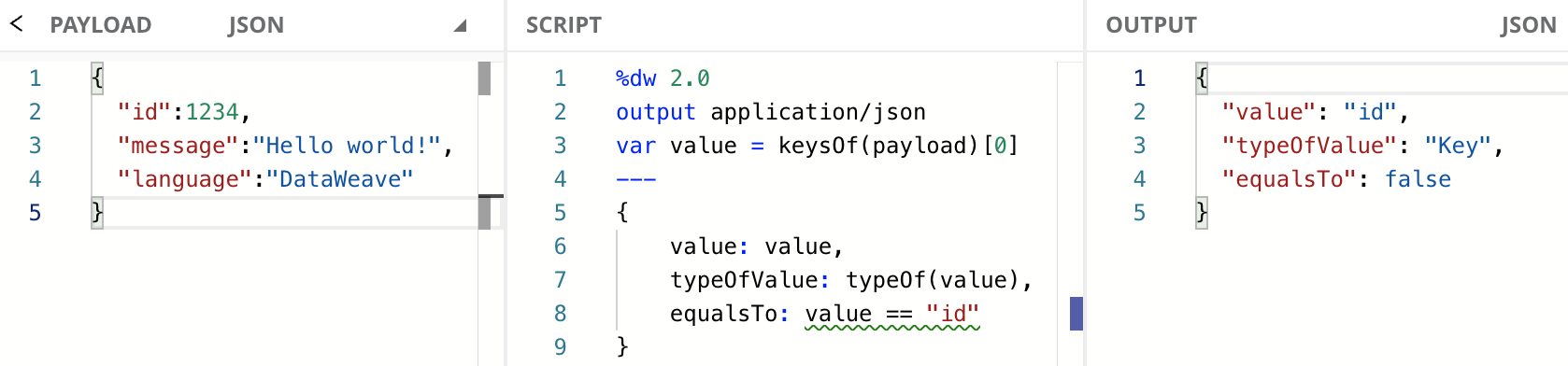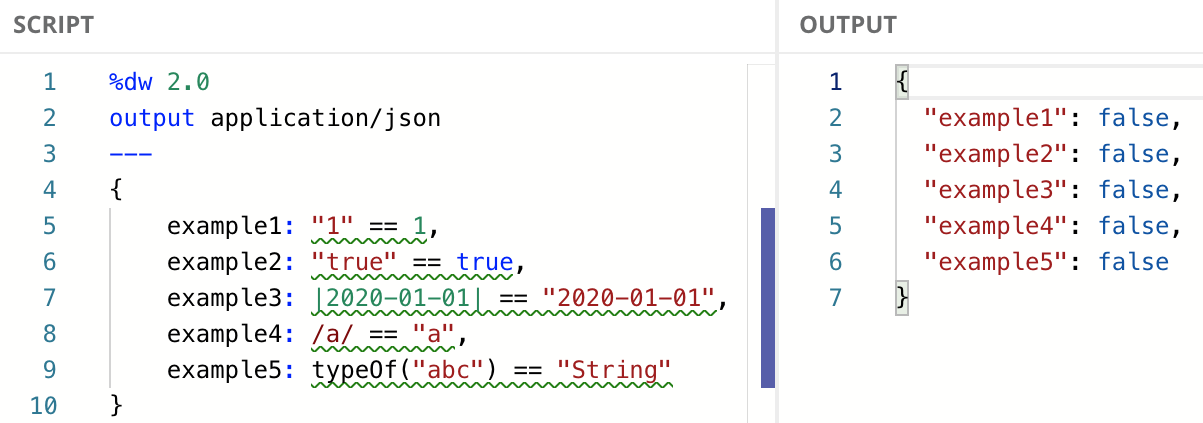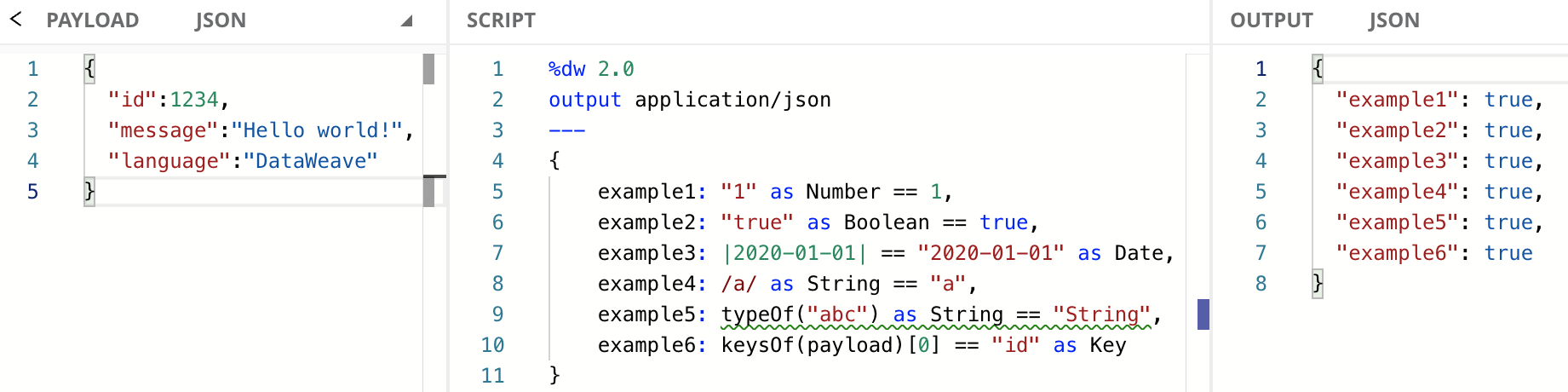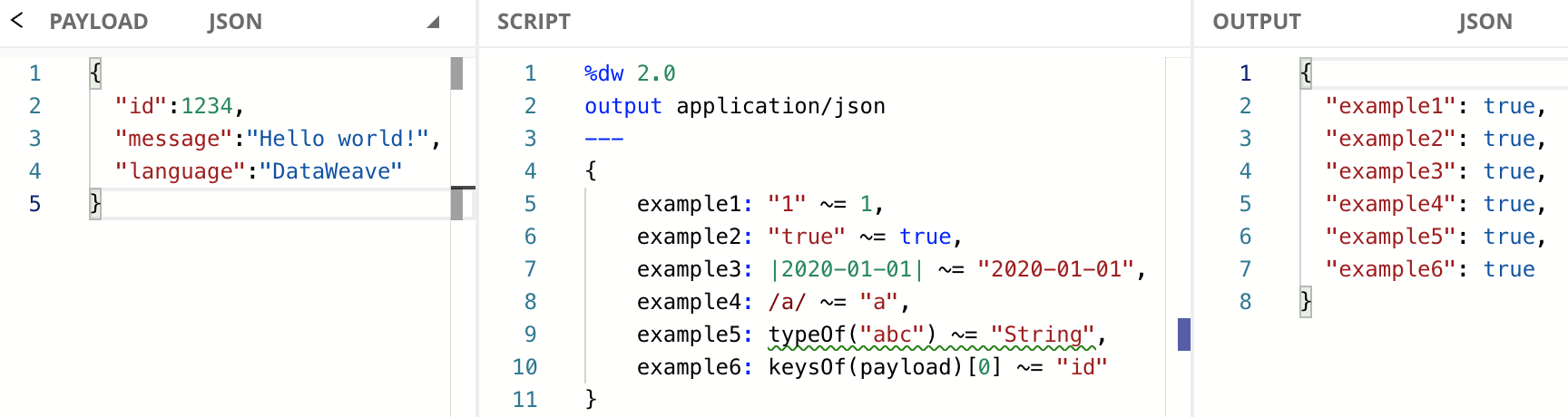# How to compare different data types in DataWeave using equality operators

10 min read | Last updated on Dec 2022

In this tutorial, you’ll learn what is the problem when using equality operators like “equal to” (`==`) or “not equal to” (`!=`) to compare values that are of different data types, like `Key == String`, `String == Number`, `Boolean == String`, and so on. You’ll learn how to cast or coerce a type and how to use the “similar to” (`~=`) operator to achieve this comparison.

You can try all of these examples with the DataWeave Playground. To learn more about it, check out this tutorial.

## Prerequisites

While not required to follow this tutorial, a good understanding of the basic DataWeave concepts would be preferred. You can check out these other tutorials if you feel a bit lost with some concepts:

## Equality Operators

The `==` operator checks if two values are equal, and part of that means checking that two values are the same type. For example, if you try to compare a Key with a String using `==`, the result will always be `false` because the types are different. Here’s an example to illustrate this with code:As you can see, we are using the `keysOf` function to generate an Array of Keys and then we are extracting only the first Key (`id`). We save this value in the `value` variable to reference it from the Object we create to output the actual value, the type of the value (`Key`), and the result of comparing this Key with the String `"id"`, which is `false`.

To learn more about the `keysOf` function, check out the documentation or this developer tutorial.

Let’s see other examples to understand how we’re going to fix these issues in this tutorial. In the following code, we can see these comparisons being made:

1. `String == Number`
2. `String == Boolean`
3. `Date == String`
4. `Regex == String`
5. `Type == String`Then how do you deal with this? You can either manually cast or coerce one of these types into the other one using the `as` keyword, or you can use the “similar to” (`~=`) operator. Whichever option you choose is ok, but we recommend using the `~=` operator whenever possible because the code is easier to understand this way. Let’s explore these different options.

## Coerce a type using `as`

You can coerce some types into a different type as long as they’re compatible. You can follow this Type Coercion Table to make sure of this. All the previous examples we used to compare are compatible types. You can choose to cast the first type into the second one, or the second one into the first one. Let’s see this in action with some examples:With this previous example, we converted one of the types to match the other one and now all our comparisons are `true` instead of `false`. This is what we did for each field:

1. Coerced `"1"` from String to Number
2. Coerced `"true"` from String to Boolean
3. Coerced `"2020-01-01"` from String to Date
4. Coerced `/a/` from Regex to String
5. Coerced `"String"` from Type to String
6. Coerced `"id"` from String to Key

## Compare the values using `~=`

This is the most recommended option to make things easier. The “similar to” operator tries to coerce one value to the type of the other when the types are different, which is what we did manually when we used `as`. Let’s follow the previous example, but instead of manually coercing the types, let’s use this operator instead of `==`.We get the same output as before, but now DataWeave is doing these coercions for us with the `~=` operator. The code looks cleaner and it’s faster to type!

You can use the `not` operator along with `~=` instead of using the “not equal to” (`!=`) operator. The `!=` operator, same as `==`, will check the types of the data. If you want to be able to compare different types, you can do something like `not String ~= Key` instead of `String != Key`, for example.

## Next Steps

In this tutorial, you learned what is the problem when using equality operators to compare values that are of different data types. You learned how to coerce a type and how to use the “similar to” (`~=`) operator to achieve this comparison.

Continue your development journey with the rest of the tutorials to become a master in DataWeave.